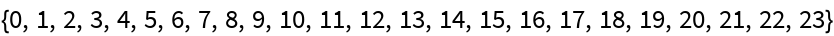# LehmerCodeFromPermutation

Contributed by: Ed Pegg Jr

Create the Lehmer code corresponding to a given permutation

 ResourceFunction["LehmerCodeFromPermutation"][perm] The Lehmer code corresponding to Lehmer code perm is created.

## Details and Options

The generating algorithm is as follows. For each number in the permutation, count how many subsequent values it exceeds.

## Examples

### Basic Examples

Generate the Lehmer code of a permutation:

 In:=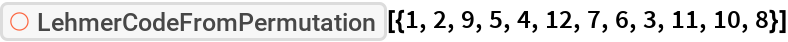Out=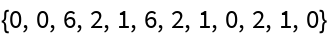### Scope

Generate the Lehmer code for a particular permutation:

 In:=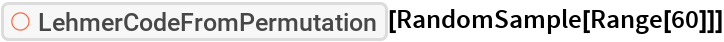Out=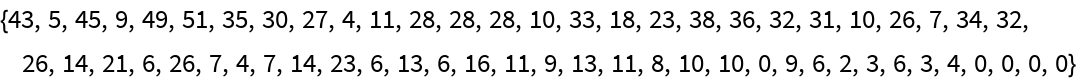### Properties and Relations

Multiplying by factorials leads to the factorial number system representation or lexicographic index of the given permutation:

 In:=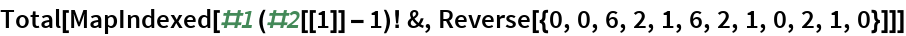Out=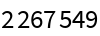The lexicographic index of a permutation works as expected:

 In:=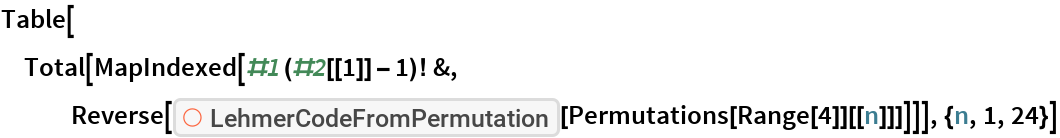Out=